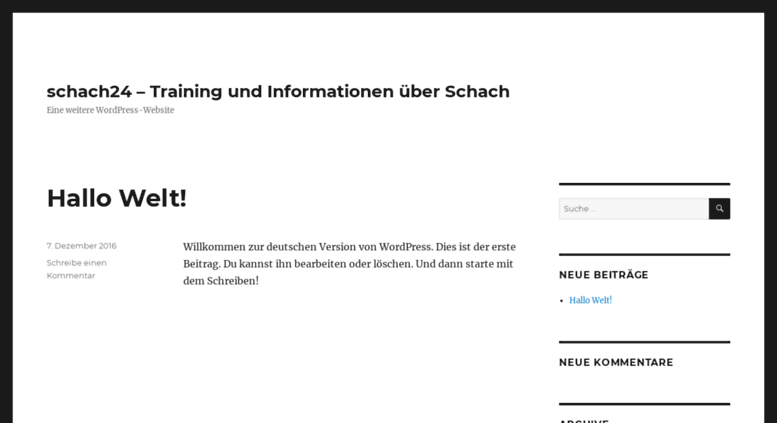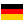# schach24.at: schach24 – Training und Informationen über Schach – Eine weitere WordPress-Website

Automarkt für Gebrauchtwagen und Neufahrzeuge aus Deutschland. Viele Auto-Angebote von Händlern und Privaten. Gebrauchtwagen von Audi bis VW..

• 59/100

Normal result
• 7

Successful tests
• 1

Failed test
• First response

448 ms

4.8 sec

• Page rendered

407 ms

5.7 sec

Visit schach24.at now to see the best up-to-date Schach 24 content and also check out these interesting facts you probably never knew about schach24.at

We analyzed Schach24.at page load time and found that the first response time was 448 ms and then it took 5.2 sec to load all DOM resources and completely render a web page. This is a poor result, as 70% of websites can load faster.

## Page optimization

•  HTML 109.6 kB Images 200.2 kB Javascripts 701.8 kB CSS 38.3 kB

In fact, the total size of Schach24.at main page is 1.0 MB. This result falls beyond the top 1M of websites and identifies a large and not optimized web page that may take ages to load. 55% of websites need less resources to load. Javascripts take 701.8 kB which makes up the majority of the site volume.

• Original 109.6 kB
• After minification 102.1 kB
• After compression 24.6 kB

HTML optimization

HTML content can be minified and compressed by a website’s server. The most efficient way is to compress content using GZIP which reduces data amount travelling through the network between server and browser. HTML code on this page is well minified. It is highly recommended that content of this web page should be compressed using GZIP, as it can save up to 85.0 kB or 78% of the original size.

• Original 200.2 kB
• After optimization 195.8 kB

Image optimization

Image size optimization can help to speed up a website loading time. The chart above shows the difference between the size before and after optimization. Schach 24 images are well optimized though.

• Original 701.8 kB
• After minification 686.6 kB
• After compression 241.7 kB

JavaScript optimization

It’s better to minify JavaScript in order to improve website performance. The diagram shows the current total size of all JavaScript files against the prospective JavaScript size after its minification and compression. It is highly recommended that all JavaScript files should be compressed and minified as it can save up to 460.1 kB or 66% of the original size.

• Original 38.3 kB
• After minification 31.0 kB
• After compression 7.6 kB

CSS optimization

CSS files minification is very important to reduce a web page rendering time. The faster CSS files can load, the earlier a page can be rendered. Schach24.at needs all CSS files to be minified and compressed as it can save up to 30.6 kB or 80% of the original size.

### Network requests diagram

• auto24.de
• geruest--0.1.137.css
• base_style--1.0000.6.css
• farben--1.0001.11.1.css
• channel1--1.0001.2.css
• jquery-1.7.2.min.js
• correction--1.00.2.js
• ct.css
• hi.js
• frame.js
• ct.js
• rc
• 1337--1.0.111.19.js
• js1--1.0.2.js
• modell--1.0.7.js
• anzeige.jpg
• sparen1.gif
• nicht_vorhanden24_klein.gif
• anzeige.jpg
• anzeige.jpg
• anzeige.jpg
• anzeige.jpg
• anzeige.jpg
• anzeige.jpg
• anzeige.jpg
• anzeige.jpg
• anzeige.jpg
• anzeige.jpg
• anzeige.jpg
• anzeige.jpg
• super-noten.jpg
• lernfox2.jpg
• peugeot-hanse.jpg
• anzeige.jpg
• auto24.de.jpg
• Startseite;sz=728x90;kw=;n3=1;n4=4;n5=3;n7=4;n8=5;n9=5;n10=5;n11=1;n12=7;n13=2;n17=4;n23=2;n39=3;n42=4;n43=3;n45=3;n46=4;n48=4;n60=4;n62=4;n72=2;n75=1;n77=1;n78=0;n79=1;n82=0;n83=1;n85=1;n86=1;n87=1;n88=1;n118=0;n119=0;d9=0;n126=0;n95=0;n127=0;n130=0;n66=1;n99=1;n97=1;n100=1;n101=1;n102=1;n105=1;n106=1;n108=1;n114=1;n74=1;n98=9;n125=1;n128=9;tile=2;dcopt=ist;ord=5120536442846060
• mtrcs_284704.js
• defaultImage.gif
• js
• osd.js
• Startseite;sz=770x250;kw=;n3=1;n4=4;n5=3;n7=4;n8=5;n9=5;n10=5;n11=1;n12=7;n13=2;n17=4;n23=2;n39=3;n42=4;n43=3;n45=3;n46=4;n48=4;n60=4;n62=4;n72=2;n75=1;n77=1;n78=0;n79=1;n82=0;n83=1;n85=1;n86=1;n87=1;n88=1;n118=0;n119=0;d9=0;n126=0;n95=0;n127=0;n130=0;n66=1;n99=1;n97=1;n100=1;n101=1;n102=1;n105=1;n106=1;n108=1;n114=1;n74=1;n98=9;n125=1;n128=9;tile=3;ord=5120536442846060
• img
• pixel
• ck-confirm
• mtrcs_284704.js
• background_angebote.jpg
• mtrcs_284704.js
• main_gif.gif
• Startseite;sz=300x250;kw=;n3=1;n4=4;n5=3;n7=4;n8=5;n9=5;n10=5;n11=1;n12=7;n13=2;n17=4;n23=2;n39=3;n42=4;n43=3;n45=3;n46=4;n48=4;n60=4;n62=4;n72=2;n75=1;n77=1;n78=0;n79=1;n82=0;n83=1;n85=1;n86=1;n87=1;n88=1;n118=0;n119=0;d9=0;n126=0;n95=0;n127=0;n130=0;n66=1;n99=1;n97=1;n100=1;n101=1;n102=1;n105=1;n106=1;n108=1;n114=1;n74=1;n98=9;n125=1;n128=9;tile=4;ord=5120536442846060
• Q116_Wu_Com_Awareness_V3_D_728x90_EN_US.jpg
• lidar.js
• sbhK2lTE.js
• cTrvNaRi.html
• express_html_inpage_rendering_lib_200_122.js
• mtrcs_284704.js
• Lrq7Jibxdtv2QOPyDSqncpDfQFNs3PE6SoUrUNh2GTU.js
• revoke
• activeview
• 300x250_PEU_Erfolgsmod_03_16_v2.jpg
• activity;src=4480062;pid=128856371;aid=302199568;ko=0;cid=69264745;rid=69144845;rv=2;met=1;×tamp=1458504230218;eid1=9;ecn1=1;etm1=0;
• lidar.js
• left_uptitle.gif
• spritecss.gif
• auto24.jpg
• Startseite;sz=120x600,160x600,200x600,300x600;kw=;n3=1;n4=4;n5=3;n7=4;n8=5;n9=5;n10=5;n11=1;n12=7;n13=2;n17=4;n23=2;n39=3;n42=4;n43=3;n45=3;n46=4;n48=4;n60=4;n62=4;n72=2;n75=1;n77=1;n78=0;n79=1;n82=0;n83=1;n85=1;n86=1;n87=1;n88=1;n118=0;n119=0;d9=0;n126=0;n95=0;n127=0;n130=0;n66=1;n99=1;n97=1;n100=1;n101=1;n102=1;n105=1;n106=1;n108=1;n114=1;n74=1;n98=9;n125=1;n128=9;tile=5;ord=5120536442846060
• revoke
• submit
• submit
• submit
• j-1575014-1259333.js
• tag.tr
• ftpagefold_v4.4.1.js
• mtrcs_284704.js
• 1259333.gif
• ft.stat
• revoke
• submit
• ga.js
• piwik.js
• beacon
• __utm.gif
• 1-2_5_16_120x600.jpg
• sbhK2lTE.js
• pixel
• beacon
• cTrvNaRi.html
• pixel
• pixel
• sd
• c.gif
• pixel

Our browser made a total of 107 requests to load all elements on the main page. We found that 0% of them (0 request) were addressed to the original Schach24.at, 12% (13 requests) were made to Cdn1.auto24.static24.at and 9% (10 requests) were made to Js2.auto24.static24.at. The less responsive or slowest element that took the longest time to load (844 ms) relates to the external source Cdn1.auto24.static24.at.

Requests

The browser has sent 103 CSS, Javascripts, AJAX and image requests in order to completely render the main page of Schach 24. We recommend that multiple CSS and JavaScript files should be merged into one by each type, as it can help reduce assets requests from 40 to 1 for JavaScripts and from 6 to 1 for CSS and as a result speed up the page load time.

40

Javascripts

52

Images

6

CSS

5

AJAX

103

All

Possible request optimization

1

Javascripts

28

Images

1

CSS

5

AJAX

68

Optimized

35

All

Normal result

Schach24.at uses IP address which is currently shared with 1 other domain. The more sites share the same IP address, the higher the host server’s workload is. It is strongly recommended that the host server should be changed or the hosting provider should be requested to give a different (separate) IP address for this domain.

Normal result

IP Trace

schach24.at

ftpserver.auto24.de

85.214.248.172

DNS records

Type Host Target/ip TTL Other
A

schach24.at

85.214.248.172 299
NS

schach24.at

ns2.netsolution24.net 3600
NS

schach24.at

ns1.netsolution24.net 3600
NS

schach24.at

ns3.netsolution24.net 3600
SOA

schach24.at

86400 Mname: ns1.netsolution24.net
Rname: andreas.auto24.de
Serial: 2016112302
Refresh: 14400
Retry: 3600
Expire: 604800
Minimum-ttl: 900

Language and encoding

Good result

Language

DEDetected

DEClaimed

Encoding

UTF-8

Claimed

Language claimed in HTML meta tag should match the language actually used on the web page. Otherwise Schach24.at can be misinterpreted by Google and other search engines. Our service has detected that German is used on the page, and it matches the claimed language. Our system also found out that Schach24.at main page’s claimed encoding is utf-8. Use of this encoding format is the best practice as the main page visitors from all over the world won’t have any issues with symbol transcription.

Similarly rated websites

HTTPS certificate

SSL Certificate

Schach24.at has no SSL certificate. Web browsing can be safer with HTTPS connection, so we suggest that it should be obtained for this site.

Normal result

Visitor World Map

The server of Schach24.at is located in Germany, but, unfortunately, we cannot identify the countries where the visitors come from and thus it’s impossible to define if the distance can potentially affect the page load time.

Good result

Ratings of similarly popular websites

Poor result

Social Sharing Optimization

Open Graph description is not detected on the main page of Schach 24. Lack of Open Graph description can be counter-productive for their social media presence, as such a description allows converting a website homepage (or other pages) into good-looking, rich and well-structured posts, when it is being shared on Facebook and other social media. For example, adding the following code snippet into HTML <head> tag will help to represent this web page correctly in social networks:

schach24.at